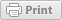Analysis of Variance for Plant Breeding

Plant Breeding and Genomics April 08, 2013Authors:

David M. Francis, The Ohio State University; Heather L. Merk, The Ohio State University; Matthew Robbins, The Ohio State University

This page provides an introduction to the analysis of variance (ANOVA), creating and interpreting simple ANOVA tables, and common applications of ANOVA to plant breeding. ANOVA has two common types of application in a plant breeding context: (1) evaluating treatment differences and (2) partitioning variance for heritability estimates.

Introduction

The analysis of variance (ANOVA) is a statistical tool that has two common applications in a plant breeding context. First, ANOVA can be used to test for differences between treatments in an experiment. Common examples of treatments are genotype, location, and variety. Second, ANOVA can be used to aid in estimates of heritability by partitioning variances. This module focuses on simple ANOVA models to evaluate differences between treatments.

Assumptions of ANOVA

Like other statistical tests, ANOVA assumes that certain assumptions are met. One of the principal assumptions of ANOVA is that the samples come from normally distributed populations, each with the same variance. In addition, it is assumed that the residuals come from a normally distributed population with equal variances (σ2). The Kruskal–Wallis test is an alternative to ANOVA when the above assumptions cannot be met.

Testing for Treatment Differences

ANOVA is a tool that can be used to test for differences among treatment means when the independent variable is categorical (e.g., genotypes could be AA, Aa, aa) and the dependent variable is continuous (e.g., yield measured in tons/acre). How does this work?

In ANOVA, the total variance of all samples is calculated. Portions of the total variance can be attributed to known causes (e.g., genotype). This leaves a residual portion of the variance that is uncontrolled or unexplained and is referred to as experimental error. Then the between-treatment variation (e.g., AA genotype variation vs. Aa genotype variation vs. aa genotype variation) is compared to the within-treatment variation (experimental error) (e.g., variation within the aa genotype) to assess whether differences in mean value between treatments are due to the treatment effects or chance.

In the simplest case, linear equations can be developed to describe the relationship between a trait and treatment. The question can then be asked, "which linear equation best fits the data for each treatment?" These linear equations take the following form:

Y = µ + f(treatment) + error

where

• Y is equal to the trait value
• µ is the population mean
• f(treatment) is a function of the treatment
• error represents the residual

In this module we provide two examples of ANOVA and sample data sets to assess differences in treatment effect. In the first example, four methods of soybean transformation are evaluated to determine whether transformation method affects expression of a stress-response gene. In the second example, two molecular markers are evaluated to determine whether genotype of each molecular marker results in differences in disease severity in a BC1 population.

Conclusion

ANOVA is a statistical tool that has applications to experiments in which we want to assess whether there is a difference in a continuous variable between treatment groups. In a plant breeding context, this page demonstrated the utility of ANOVA in gene expression studies and molecular marker analysis.

Many statistics textbooks provide a good discussion of theory and applications of ANOVA. A few examples are listed below.

• Clewer, A. G., and D. H. Scarisbrick. 2001. Practical statistics and experimental design for plant and crop science. John Wiley & Sons Ltd., New York.
• Steel, R. G. D., J. H. Torrie, and D. A. Dickey. 1997. Principles and procedures of statistics a biometrical approach. The McGraw-Hill Companies, Inc., New York.

The following videos provide detailed instructions for calculating components of ANOVA tables (ANOVA1 and 2) and hypothesis testing (ANOVA3).

Funding Statement

Development of this page was supported in part by the National Institute of Food and Agriculture (NIFA) Solanaceae Coordinated Agricultural Project, agreement 2009-85606-05673, administered by Michigan State University. Any opinions, findings, conclusions, or recommendations expressed in this publication are those of the author(s) and do not necessarily reflect the view of the United States Department of Agriculture.

PBGworks 650

Welcome

This is where you can find research-based information from America's land-grant universities enabled by eXtension.orgThis work is supported by the USDA National Institute of Food and Agriculture, New Technologies for Ag Extension project.